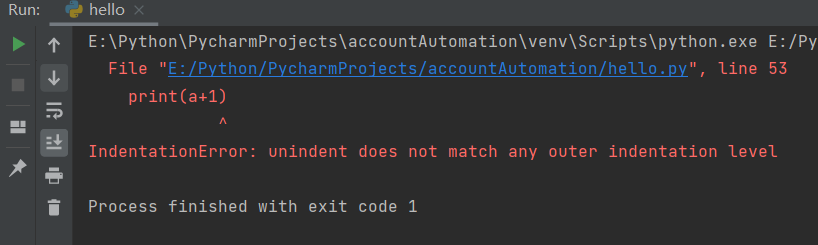墨滴Daner

2021/06/02  阅读：73  主题：凝夜紫

# python入门——语法基础

## 一行写不下，我换行怎么换，多行代码

• 可能还不理解我写的是什么，不要紧，先看规则
• 让代码换行显示加\
• 带括号[]{}()的直接换行不用连接符号

## 把多行语句写在一行

• 加分号 ; 如果你不加还是会出语法错误
``word_zero = ' 'word_one = 'hello'print(word_zero);print(word_one)``

## 引号

``word_zero = ' 'word_one = 'hello'word_two = "your's world"word_three = '''你好世界你好python好好学习天天向上'''print(word_zero)print(word_one)print(word_two)print(word_three)``
• 单引号 字符串
• 双引号 字符串包含单引号，不包含也可以写双引号
• 三个引号（单或者双） 段落，多行字符的段落

## 注释

``#这是注释，不被python解释器执行的#print('hello python')print('hello python')``

## 等待用户输入

``input('请输入一些字符，按下回车键Enter退出\n')``

## print输出

``x= 'Aa'y= 'Bb'X= 'Xx'# 输出在一行 无缝连接print(x+y+X)# 分隔下输出的结果方便查看print('-' * 20)# 输出在一行 空格分隔print(x, y, X)print('-' * 20)# 换行输出 # end=''参数可以使得print不换行print(x, end='')print(y)print(X)``

## 行和缩进

python不像Java使用{}来控制代码逻辑，类，方法，用缩进来控制逻辑

``a = 1if a == 1:    print('变量a是1')else:    print('变量a不是1')``
``a = 1if a == 1:    print('变量a是1') print(a+1)else:    print('变量a不是1')``正确缩进代码可读性也强，即便Java有{}也建议在编码时保持良好的缩进，更何况python缩进不对都没法正常按逻辑干活了Daner

2021/06/02  阅读：73  主题：凝夜紫